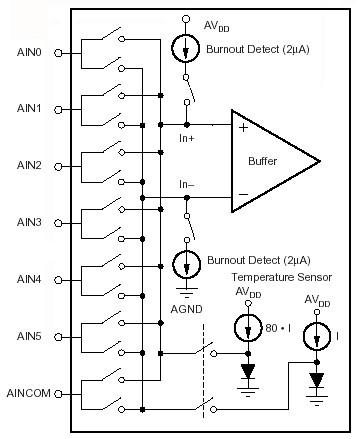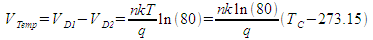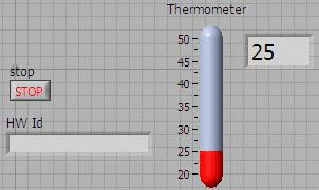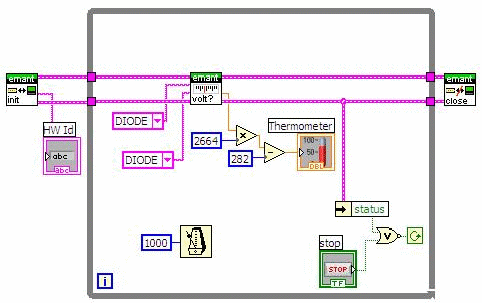The following information is for the EMANT300, EMANT380

Measure Temperature using EMANT3X0 onboard Temperature Sensing DiodesThe EMANT3X0 has a pair of temperature sensing diodes D1 and D2, as shown in Figure 1. The differential inputs of the ADC converter are selectable via input multiplexers. The ADC inputs can be set to connect to the temperature diode outputs. The differential voltage of the diode pair provides a temperature reading.

Equation 1 shows the PN junction ideal diode equation. ID is from a constant current source.where
VD - Diode voltage
n - Diode emission coefficient
k - Boltzman constant
T - Temperature in deg K
q - Charge of an electron
ID - Diode current
IS - Diode saturation current

To eliminate IS, a differential measurement is used. The diode voltages for D1 and D2 in Figure 1 are VD1 and VD2, respectively. The difference of the diode voltages is VTemp. The constant current applied to the diodes, ID1 and ID2, is designed such that ID2 = 80*ID1. The two diodes are also designed such that diode saturation currents, IS, of the two diodes are identical. Therefore the diode pair output voltage VTemp is proportional to the absolute temperature T which is then converted to TC where TC is the temperature in oCAfter linearising, the temperature / voltage relationship can be simplified to

TC = α * VTemp - 282.14

One value of α that gives good results is 2664.7. The value of α can vary from part to part and is determined from experimental data and calibration.

Heat generated by sensors when power is applied causes self-heating. This affects the calibration coefficient. The device heat dissipation is proportional to the power supply voltage, operation frequency, and on-chip operating features.

The LabVIEW VI that reads the voltage across the diodes, converts the voltage to temperature is called EMANT300 Example Diode Temperature.VI Please note that for the ADC voltage reference to work, REFIN- pin 4 of the DB25 connector needs to be connected to AGND. If you have connected the Light Application Adaptor, REFIN- is already connected to AGND.

Front PanelBlock Diagram